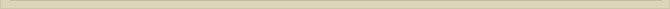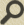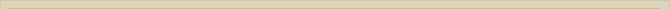## The Transformation to the third Quantum Dimension (Bohr-distance)

When you are interested in physics you must read “Unbelievable“!

We demonstrated that with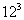/2 point-volumes we can create a perfect “circle” with 864 triangles OAB. Point O (figure 4) is the center of the created Compton quantum circle (Rc).  Between and Rc the quantum space is imperfect. The “bulbs” with radius QD cannot fill up spherical space homogeneously.

The “perfect” geometry is created at Rc. For the observer in O it is not possible to observe perfect circles all around (no perfect bulb possible when space is filled with point-volumes).

The orientation of the two possible circles Rc is not fixed.

With the “perfect” two-dimensional circle Rc we are able to create a perfect bulb shell tunnel with diameter Rc at the distance*Rc; the Bohr-distance.Figure 6.  Compton circles creating the 3rd  quantum dimension.

With/2 circles Rc (two circles each) we are able, in conjunction with the creation of the Compton-circle, to create two “perfect” bulb shell tunnels with radius Rc at the Bohr-distance; the beginning of the 3rd quantum dimension.

The ratio between the distances Rb and Rc should be.

We have observed that the ratio between the Bohr-radius and the Compton-radius is:

The Bohr/Compton distance ratio appears to be 10.8674 times larger than the ratio Rc/QD or the Rydberg/Bohr ratio.

We must however not forget that the situation at 2-QD is not the same as the 1-QD level or the 3-QD level. We have seen that a mathematical correction from 1-QD to 2-QD with the factor 2pi was necessary. We now compare the 3rd dimension of the Bohr-bulb with 2nd dimension of the Compton-plate. A mathematical correction is necessary.

The factor 10.8674 is the correction factor from the 2-QD to the 3-QD. From the 1st to the 2nd QD the correction factor is 2π. This transformation explains the origin of the mathematical natural constant π. Is it a coincidence that the other mathematical natural constant e can be found in 10.8674, becauseand the difference is therefore only 0.05%?  **

So both mathematical natural constants may well originate from the dimension transfer from the point-volume to three-dimensional space.

After the dimension correction we have also the ratio Rb/Rc=.

The total mathematical correction from the point-volume (1st dimension) to our 3rd dimension is: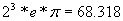.

(** When we correct for neglecting the factor (1+Me/Mp)=1.0005446, when equation 5a was derived from 5, the deviation factor with 4*e is less than 2.10^-5)

Next chapter: The 12 Ionization Levels of the Atom